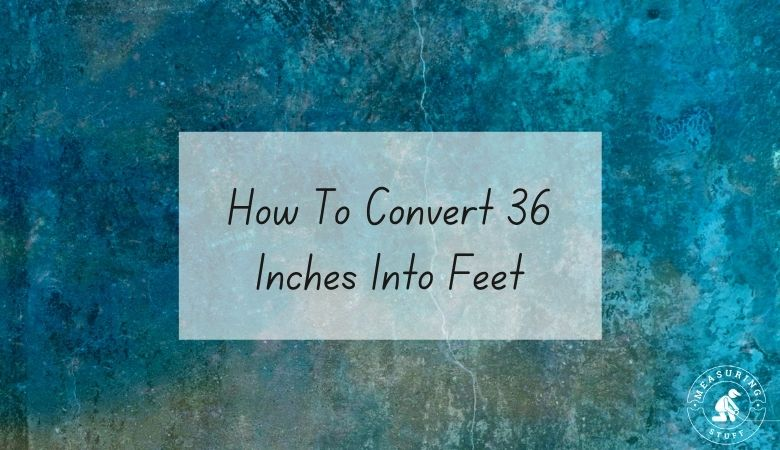# How To Convert 36 Inches Into Feet

Learning how to convert 36 inches into feet is quite simple.

There are 3 feet in 36 inches.

In order to find out how many feet are in a certain number of inches, you would need to divide the number of inches by 12.

There are 12 inches in 1 foot, so dividing any number of inches by 12 will give you the answer in feet.

The formula is:

# of feet = # of inches / 12

For example, if you want to know how many feet are in 36 inches, divide 36 by 12.

The answer, which is 3, tells you that there are that many feet in 36 inches.

If you want to reverse the question and figure out how many inches can be made from a certain number of feet, you would multiply the number of feet by 12.

For example, if you have 3 feet, you can multiply it by 12 to get 36.

This means that there are 36 inches in 3 feet.

If you don’t feel like doing the math, use our online conversion calculator below to convert different units of measurements.

## Inches into feet conversion calculator

Check out all our conversion calculators here.

Other articles on our site using inches measurements include:

You can also convert 36 inches into other units of measurements.

36 inches = 91.44 centimeters

36 inches = 1 yard

36 inches = 914.4 millimeters

## Summary

You should now be able to convert 36 inches into feet easily. Feel free to share our page on social media and save our website in your bookmarks for easy and quick reference.